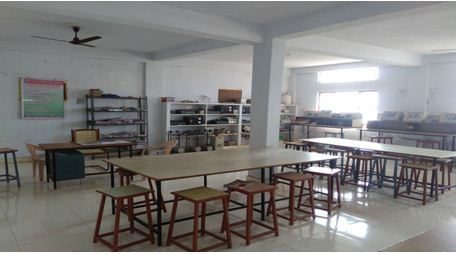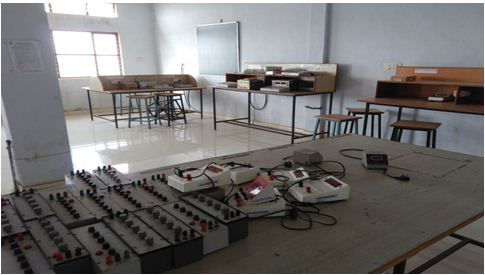### ELECTRICAL MACHINES LAB – I

Course Objectives:

1) To expose the students to the operation of DC Generator

2) To expose the students to the operation of DC Motor.

3) To examine the self excitation in DC generators.

The following experiments are required to be conducted compulsory experiments:

1. Magnetization characteristics of DC shunt generator. Determination of critical field resistance and critical speed.
2. Load test on DC shunt generator. Determination of characteristics.
3. Load test on DC series generator. Determination of characteristics
4. Load test on DC compound generator. Determination of characteristics.
5. Hopkinson’s test on DC shunt machines. Predetermination of efficiency.
6. Fields test on DC series machines. Determination of efficiency.
7. Swinburne’s test and speed control of DC shunt motor. Predetermination of efficiencies.
8. Brake test on DC compound motor. Determination of performance curves.

In addition to the above eight experiments, at least any two of the experiments from the following list are required to be conducted:

1. Brake test on DC shunt motor. Determination of performance curves.
2. Retardation test on DC shunt motor. Determination of losses at rated speed.
3. Separation of losses in DC shunt motor### Electrical Machines-II Lab

Course educational objectives:

On completion of this Subject/Course the student shall be able to

1. To prepare the students to have a basic knowledge of transformers.
2. To prepare the students to have a basic knowledge of induction motors.
3. To prepare the students to have a basic knowledge of alternators.
4. To design a practical transformer.

The following experiments are required to be conducted as compulsory experiments

1. O.C. & S.C. Tests on Single phase Transformer
2. Sumpner’s test on a pair of single phase transformers
3. No-load & Blocked rotor tests on three phase Induction motor
4. Regulation of a three –phase alternator by synchronous impedance&m.m.f. methods
5. V and Inverted V curves of a three—phase synchronous motor.
6. Equivalent Circuit of a single phase induction motor
7. Determination of Xd and Xq of a salient pole synchronous machine
8. Load test on three phase Induction Motor

In addition to the above experiments, at least any two of the following experiments are   required to be conducted from the following list

1. Separation of core losses of a single phase transformer
2. Efficiency of a three-phase alternator
3. Parallel operation of Single phase Transformers
4. Regulation of three-phase alternator by Z.P.F. and A.S.A methods
5. Heat run test on a bank of 3 Nos. of single phase Delta connected transformers
6. Measurement of sequence impedance of a three-phase alternator.
7. Vector grouping of Three Transformer
8. Scott Connection of transformerPOWER ELECTRONICS LAB

Course Description & Objectives:

This course aims at obtaining characteristics of power electronic devices. To understand the commutation techniques used in power electronics circuits and to test different power electronics converters.

List of Experiments:

1. Study of Characteristics of SCR, MOSFET & IGBT
2. Gate firing circuits for SCR’s
3. Single Phase AC Voltage Controller with R and RL Loads
4. Single Phase fully controlled bridge converter with R and RL loads
5. Forced Commutation circuits ( Class A, Class B, Class C, Class D & Class E)
6. DC Jones chopper with R and RL Loads
7. Single Phase Parallel, inverter with R and RL loads
8. Single Phase Cycloconverter with R and RL loads
9. Single Phase Half controlled converter with R load
10. Three Phase half controlled bridge converter with R-load
11. Single Phase series inverter with R and RL loads
12. Single Phase Bridge converter with R and RL loads
13. Single phase dual converter with RL loads
14. Operation of MOSFET based chopper

Any two simulation experiments with PSPICE/PSIM

1. Single phase full converter using RLE loads and single phase ac voltage controller using RLE loads.
2. Resonant pulse commutation circuit and Buck chopper.
3. Single-phase inverter with PWM control.Electric Circuits & Simulation Lab

COURSE OBJECTIVES:

This course enables the students to:

1) Construct and verify various electrical circuits applying network theorems.

2) Learn different locus diagrams for various electrical circuits like RL, RC and RLC.

3) Analyze different models of electric circuits through simulation by using PSPICE and     MATLAB software.

4) Understand the concepts of resonating conditions in series and parallel circuits.

5) Evaluate the various electrical and electronic parameters using two –port networks.

Note: Eight experiments are to be conducted from PART-A and any Two from PART-B

PART-A: ELECTRIC CIRCUITS

1) Verification of Thevenin’s and Norton’s Theorems.

2) Verification of Superposition theorem and Maximum Power Transfer Theorems.

3) Verification of RMS value of complex wave.

4) Verification of compensation theorem.

5)) Verification of Reciprocity, Millmann’s Theorems.

6) Locus diagram of RL and RC series circuits

7) Series and Parallel Resonance.

8) Determination of Self, Mutual Inductances and Coefficient of coupling.

9) Determination of Z and Y Parameters.

10) Determination of Transmission and hybrid parameters.

11) Measurement of Active Power for Star and Delta connected balanced loads.

12) Measurement of Reactive Power for star and delta connected balanced loads.

13) Measurement of 3-phase active Power by two Wattmeter Method for Unbalanced loads.

PART-B: PSPICE SIMULATION

1) Simulation of DC Circuits

2) DC Transient response

3) Mesh Analysis

4) Nodal AnalysisELECTRICAL MEASUREMENTS AND INSTRUMENTATION LABORATORY

Course objectives

• To calibrate LPF Watt Meter, energy meter, P.F Meter using electro dynamo meter type instrument as the standard instrument
• To determine unknown inductance, resistance, capacitance by performing experiments on D.C Bridges &A.C Bridges
• To determine three phase active & reactive powers using single wattmeter method practically
• To determine the ratio and phase angle errors of current transformer and potential transformer.

The following experiments are required to be conducted as compulsory experiments:

1. Calibration and Testing of single phase energy Meter
2. Calibration of dynamometer power factor meter
3. Crompton D.C. Potentiometer – Calibration of PMMC ammeter and PMMC voltmeter
4. Kelvin’s double Bridge – Measurement of resistance – Determination of Tolerance.
5. Dielectric oil testing using H.T. testing Kit
6. Schering bridge & Anderson bridge
7. Measurement of 3 phases reactive power with single-phase wattmeter.
8. Measurement of parameters of a choke coil using 3 voltmeter and 3 ammeter methods.

In addition to the above eight experiments, at least any two of the experiments from the following list are required to be conducted:

1. Calibration LPF wattmeter – by Phantom testing
2. Measurement of 3 phase power with single watt meter and 2 No’s of C.T.
3. C.T. testing using mutual Inductor – Measurement of % ratio error and phase angle of given C.T. by Null method.
4. P.T. testing by comparison – V.G. as Null detector – Measurement of % ratio error and phase angle of the given P.T.
5. LVDT and capacitance pickup – characteristics and Calibration
6. Resistance strain gauge – strain measurements and Calibration
7. Transformer turns ratio measurement using a.c. bridge
8. Measurement of % ratio error and phase angle of given C.T. by comparison.Control Systems

Course Objectives:

1) To understand the different ways of system representations such as Transfer function representation and state space representations and to assess the system dynamic response.

2) To assess the system performance using time domain analysis and methods for improving it

3) To assess the system performance using frequency domain analysis and techniques for improving the performance

4) To design various controllers and compensators to improve system performance

The following experiments are required to be conducted compulsory experiments:

1) Time response of Second order system

2) Characteristics of Synchros

3) Programmable logic controller – Study and verification of truth tables of logic gates, simple Boolean expressions, and application of speed control of motor.

4) Effect of feedback on DC servo motor

5) Transfer function of DC motor

6) Effect of P, PD, PI, PID Controller on a second order systems

7) Lag and lead compensation – Magnitude and phase plot

8) Transfer function of DC generator

9) Temperature controller using PID

10) Characteristics of magnetic amplifiers

11) Characteristics of AC servo motor.

In addition to the above eight experiments, at least any two of the experiments from the following list are required to be conducted

1) PSPICE simulation of Op-amp based integrator and differentiator circuits.

2) Linear system analysis (time domain analysis, error analysis) using MAT LAB.

3) Stability analysis (bode, root locus, nyquist) of linear time invariant system using MAT LAB.

4). State space model for classical transfer function using suitable MATLAB -Verification.### Department of Electrical and Electronics Engineering Infrastructure

Financial and Physical Resources

S.No Name of the Lab     Area(Sqmt)Expenditure Rs.
1.Electrical Machines Lab - I  75    Rs. 6,28,716/-
2.Electrical Machines Lab - II  75Rs.5,32,180/-
3.Electrical Circuits and Simulation 75Rs.1,43,335/-
4.Control Systems Lab  75Rs.1,59,050/-
5Power Electronics  Lab 75Rs.2,24,235/-
6.Electrical Measurements Lab 75Rs. 2,08,948/-
7.Electrical and Electronics Lab  75Rs.2,44,121/-
8 Total Rs.525Rs. 19,31,637